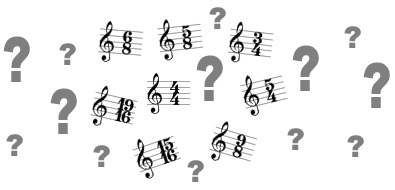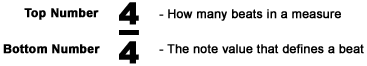• Lessons
• Articles

# Time Signatures - How They Work

Sean Conklin 456 lessons4/4? 7/8? 3/4? 6/8? 19/16? 9/8? What do all these numbers mean!?

Ever wonder what those fraction looking things are at the beginning of every piece of music? And what do those numbers mean anyway? What are they for? Well, have no fear my guitar-playing friend. Time signatures aren't as complicated as they might seem.

Every piece of music has a time signature that indicates how to recognize the beats in a measure. Why is this important? Because without time signatures, we'd have no way of knowing how to associate timing with the music. Let's get to the specifics...

Time signatures look exactly like a fraction, where there is a top number and a bottom number. Both of the numbers have very significant meanings. Let's take a look...So now that we know what each number represents, we need to know how those numbers work together. In the above image, we see a 4/4 time signature. So if we look just at the top number, we can see that there are 4 beats of some kind. Then, the next thing we need to figure out is what kind of beats by looking at the bottom number. So if we look at the bottom number, we see a 4. That means the beats will be represented by quarter notes. Now that we know what the top and bottom numbers mean, we can conclude that there are 4 quarter notes in a 4/4 time signature.

What if the bottom number is something other than 4? Let's look...

If the bottom number is:
4, then it represents quarter notes
8, then it represents eighth notes
16, then it represents sixteenth notes

Now, let's go over some examples of different time signatures and how we would interpret them...

4/4 = 4 quarter notes in a measure
6/8 = 6 eighth notes in a measure
5/4 = 5 quarter notes in a measure
15/16 = 15 sixteenth notes in a measure
9/8 = 9 eighth notes in a measure
19/16 = 19 sixteenth notes in a measure
3/4 = 3 quarter notes in a measure

Starting to see how it works now? So anytime you see a time signature, just say whatever the top number is, then follow that with whatever kind of note value the bottom number represents. So for example, if you saw a 5 on top of an 8, you can immediately conclude "5 eighth notes" in a measure.

Alright, good job! That should get you started with figuring out how time signatures work. If you ever have any questions, feel free to ask in the forums.

Lastly, if you'd like to learn more, feel free to check out my in-depth time signature study in this tutorial.

Send this to a friend